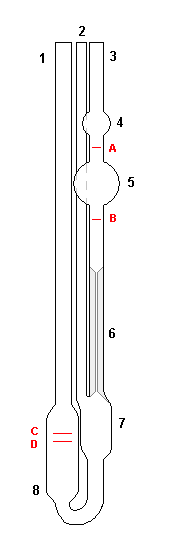# How to Calculate Molecular Weight of Polymer

Polymers contain a wide range of chains with different molecular weight, unlike many other substances. Thus, we cannot calculate the exact molecular weight of a polymer; instead, we calculate the average molecular weight of a polymer. It is very much important to determine the average molecular weight of a polymer as their properties depend on it. For example, a polymer with a large average molecular weight would behave in an entirely different manner to a polymer with a small average molecular weight. There are various approaches to calculating the average molecular weights of polymers including viscometry, osmometry, size-exclusion chromatography, ultracentrifugation, light scattering, end-group analysis and turbidimetric titration.

1. How to Calculate Molecular Weight of Polymer?
– Number-average molecular weight
– Weight-average molecular weight

2. What is Viscometry?

3. What is Ultracentrifugation?

## How to Calculate Molecular Weight of Polymer

There are two common ways to calculate the average molecular weight of polymers if the molecular weight distributions are known.

### Number-average Molecular Weight

The first method is the number-average molecular weight (n), which is found by the equation;

n= Σ xi Mi

Mi is the mean molecular of the size range i, and xi is the fraction of the total number of chains within the given range.

### Weight-average Molecular Weight

The second method is the weight-average molecular weight (Ḿw), found by equation;

w= Σ wi Mi

Mi is the mean molecular of the size range i, and wi is the weight fraction of the molecules within the given range.

## What is Viscometry

The most common technique that is used to determine the average molecular weight of a polymer is the viscometry, where Ubbelohde viscometer is employed. In this method, the polymer should be in liquid form; if not, it has to be dissolved using a solvent with a known concentration. According to Mark-Houwink equation, the molecular weight can be calculated if the viscosity (η) is known.

[η] = K M a    ………………1

K and a are known constants that depend on the solvent, the type of polymer and the temperature.

The value of [η] can be determined by plotting ((η- η0)/ η0c) as a function of c, where c is the concentration of the polymer solution. In order to determine the η using viscometer, first, a series of solutions of polymers should be made. Then the efflux time has to be determined for each solution (t). According to Huggins equation,

(t – t0) / t0c = (ηηo)/ η0c  ……………2

t0 is the efflux time of the pure solvent without polymer. By using the above 1 and 2 equation, we can determine the average viscosity of a polymer.Ubbelohde viscometer

## What is Ultracentrifugation

In this method, the average molecular weight of a polymer is determined by measuring rates of sedimentation. Sedimentation rate of particles is usually very slow. In order to accelerate the process, we can use an ultracentrifuge. The average molecular weight of a polymer can be determined by the following equation;

M= S R T / (1- ρνs) D

M is the molecular weight of the sample, R is the universal gas constant, T is the temperature, ρ is the solution density,  νs is the specific volume of the solute, S is the sedimentation constant, and D is the diffusion coefficient.

S has to be determined first using the equation below.

S= s / r ω2

s is the speed, r is the radius and ω is the angular velocity.

In addition to these main techniques, chromatography, osmometry, light scattering, end-group analysis, and turbidimetric titration methods are also applied to determine molecular weights of polymers. However, the most commonly used and the simplest technique is the viscometry.

References

Stuart, B. H. (2008).Polymer analysis (Vol. 30). John Wiley & Sons.

Image Courtesy:

“Ubbelohde lepkosciomierz” By Warczp – Own work (CC BY-SA 3.0) via Commons Wikimedia